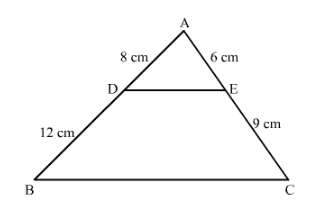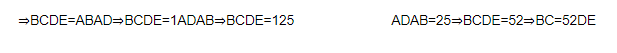# D and E are the points on the sides AB and AC respectively of a ∆ABC such that: AD = 8 cm,

Question:

D and E are the points on the sides AB and AC respectively of a ∆ABC such that: AD = 8 cm, DB = 12 cm, AE = 6 cm and CE = 9 cm, Prove that BC = 5/2 DE.

Solution:

It is given that $A D=8 \mathrm{~cm}, D B=12 \mathrm{~cm}, A E=6 \mathrm{~cm}$ and $C E=9 \mathrm{~cm}$.We have to prove that $B C=\frac{5}{2} D E$

Since clearly $\frac{A D}{A B}=\frac{A E}{A C}=\frac{2}{5}$

Also, $\angle A$ is common in $\triangle A B C$ and $\triangle A D E$

So $\triangle A D E \sim \triangle A B C$ (SAS Similarity)